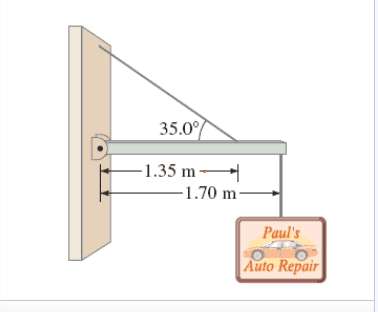# Sign hanging at angle

A shop sign weighing 215 N hangs from the end of a uniform 155-N beam.

Tension in supporting wire= 642 N
Horizontal force exerted by the hinge= 526N

Find the vertical force exerted by the hinge on the beam at the wallFy= Fhy + Ft * sin() - mg - Mg =0

My attempt:
Fhy= -(Ft *sin() - mg - Mg)
=-(642*sin(35) - 155 - 215)
= 1.76 N

My answer is incorrect, but I don't know what I'm doing wrong.

#### Attachments

Last edited:

haruspex
Homework Helper
Gold Member
2020 Award
A shop sign weighing 215 N hangs from the end of a uniform 155-N beam.

Tension in supporting wire= 642 N
Horizontal force exerted by the hinge= 526N

Find the vertical force exerted by the hinge on the beam at the wall

Fy= Fhy + Ft * sin() - mg - Mg =0

My attempt:
Fhy= -(Ft *sin() - mg - Mg)
=-(642*sin(35) - 155 - 215)
= 1.7 N

My answer is incorrect, but I don't know what I'm doing wrong.
How did you get that tension? I get rather less.

How did you get that tension? I get rather less.
I used the formula Ft= (mg length/2 + Mglength) / (length*sin(35))

haruspex
Homework Helper
Gold Member
2020 Award
I used the formula Ft= (mg length/2 + Mglength) / (length*sin(35))
The cable is not attached to the end of the rod.

The cable is not attached to the end of the rod.

It doesn't matter where on the rod it's attached to in this scenario. It goofed me up at first because I thought it did matter when it actually did not. This is the correct tension.

haruspex
Homework Helper
Gold Member
2020 Award
It doesn't matter where on the rod it's attached to in this scenario. It goofed me up at first because I thought it did matter when it actually did not. This is the correct tension.
For the torque, it matters.
However, you are right that the tension is 642N, I made a mistake.

haruspex
Homework Helper
Gold Member
2020 Award
So, now I wonder whether your 1.7 is not quite accurate enough. I get exactly 5/3N. (There's a lot of cancellation, and angle turns out not to matter.)

So, now I wonder whether your 1.7 is not quite accurate enough. I get exactly 5/3N. (There's a lot of cancellation, and angle turns out not to matter.)
I get exactly 1.7639278626, rounded to 3 sig figs is 1.76 N which is incorrect.

haruspex